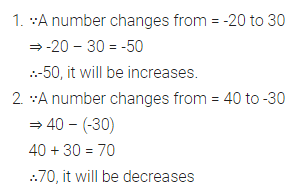# Selina Concise Mathematics Class 7 ICSE Solutions Chapter 1 Integers

## Selina Concise Mathematics Class 7 ICSE Solutions Chapter 1 Integers

Selina Publishers Concise Mathematics Class 7 ICSE Solutions Chapter 1 Integers

### Integers Exercise 1A – Selina Concise Mathematics Class 7 ICSE Solutions

Question 1.
Evaluate:

1. 427 x 8 + 2 x 427
2. 394 x 12 + 394 x (-2)
3. 558 x 27 + 3 x 558

Solution:Question 2.
Evaluate:

1. 673 x 9 + 673
2. 1925 x 101 – 1925

Solution:Question 3.
Verify:

1. 37 x {8 +(-3)} = 37 x 8 + 37 x – (3)
2. (-82) x {(-4) + 19} = (-82) x (-4) + (-82) x 19
3. {7 – (-7)} x 7 = 7 x 7 – (-7) x 7
4. {(-15) – 8} x -6 = (-15) x (-6) – 8 x (-6)

Solution: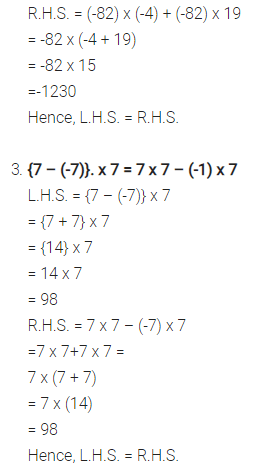Question 4.
Evaluate:

1. 15 x 8
2. 15 x (-8)
3. (-15) x 8
4. (-15) x -8

Solution: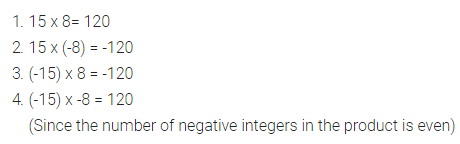Question 5.
Evaluate:

1. 4 x 6 x 8
2. 4 x 6 x (-8)
3. 4 x (-6) x 8
4. (-4) x 6 x 8
5. 4 x (-6) x (-8)
6. (-4) x (-6) x 8
7. (-4) x 6 x (- 8)
8. (-4) x (-6) x (-8)

Solution:Question 6.
Evaluate:

1. 2 x 4 x 6 x 8
2. 2 x (-4) x 6 x 8
3. (-2) x 4 x (-6) x 8
4. (-2) x (-4) X 6 x (-8)
5. (-2) x (-4) x (-6) x (-8)

Solution:Question 7.
Determine the integer whose product with ‘-1’ is:

1. -47
2. 63
3. -1
4. 0

Solution: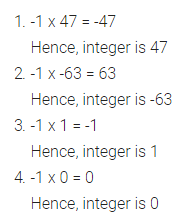Question 8.
Eighteen integers are multiplied together. What will be the sign of their product, if:

1. 15 of them are negative and 3 are positive?
2. 12 of them are negative and 6 are positive?
3. 9 of them are positive and the remaining are negative?
4. all are negative?

Solution: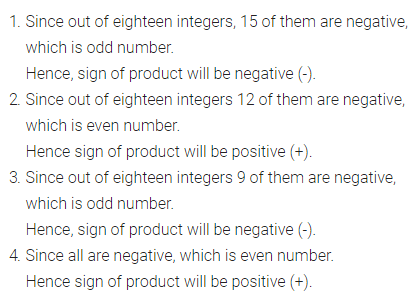Question 9.
Find which is greater?

1. (8 + 10) x 15 or 8 + 10 x 15
2. 12 x (6 – 8) or 12 x 6 – 8
3. {(-3) – 4} x (-5) or (-3) – 4 x (-5)

Solution: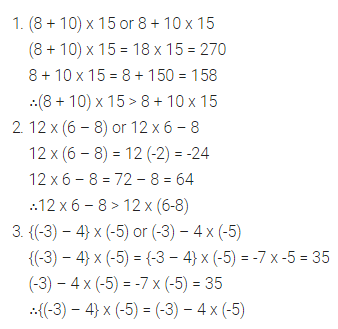Question 10.
State, true or false :

1. product of two integers can be zero.
2. product of 120 negative integers and 121 positive integers is negative.
3. a x (b + c) = a x b + c
4. (b – c) x a=b – c x a

Solution:### Integers Exercise 1B – Selina Concise Mathematics Class 7 ICSE Solutions

Question 1.
Divide:
(i) 117 by 9
(ii) (-117) by 9
(iii) 117 by (-9)
(iv) (-117) by (-9)
(v) 225 by (-15)
(vi) (-552) ÷ 24
(vii) (-798) by (-21)
(viii) (-910) ÷ – 26

Solution :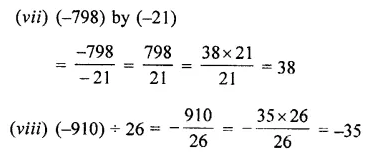Question 2.
Evaluate:
(i) (-234) ÷ 13
(ii) 234 ÷ (-13)
(iii) (-234) ÷ (-13)
(iv) 374 ÷ (-17)
(v) (-374) ÷ 17
(vi) (-374) ÷ (-17)
(vii) (-728) ÷ 14
(viii) 272 ÷ (-17)

Solution:Question 3.
Find the quotient in each of the following divisions:
(i) 299 ÷ 23
(ii) 299 ÷ (-23)
(iii) (-384) ÷ 16
(iv) (-572) ÷ (-22)
(v) 408 ÷ (-17)

Solution: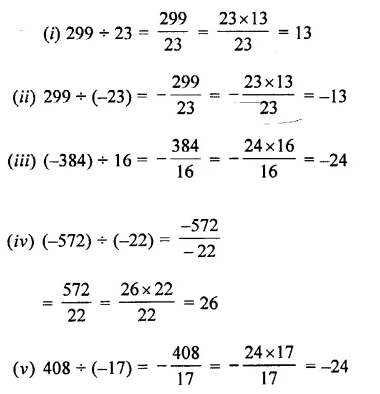Question 4.
Divide:
(i) 204 by 17
(ii) 152 by-19
(iii) 0 by 35
(iv) 0 by (-82)
(v) 5490 by 10
(vi) 762800 by 100

Solution: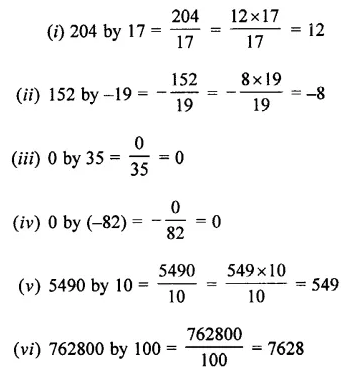Question 5.
State, true or false :

1. 0 ÷ 32 = 0
2. 0 ÷ (-9) = 0
3. (-37) ÷ 0 = 0
4. 0 ÷ 0 = 0

Solution: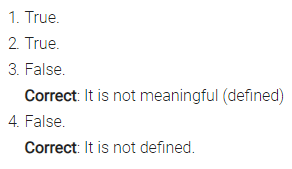Question 6.
Evaluate:
(i) 42 ÷ 7 + 4
(ii) 12+18 ÷ 3
(iii) 19 – 20 ÷ 4
(iv) 16 – 5 x 3+4
(v) 6 – 8 – (-6) ÷ 2
(vi) 13 -12 ÷ 4 x 2
(vii) 16 + 8 ÷ 4- 2 x 3
(viii) 16 ÷ 8 + 4 – 2 x 3
(ix) 16 – 8 + 4 ÷ 2 x 3
(x) (-4) + (-12) ÷ (-6)
(xi) (-18) + 6 ÷ 3 + 5
(xii) (-20) x (-1) + 14 – 7

Solution: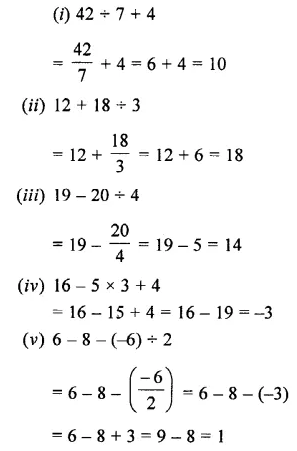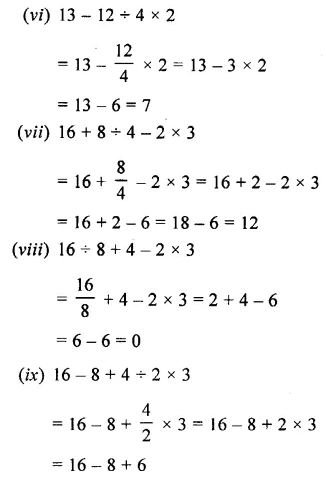### Integers Exercise 1C – Selina Concise Mathematics Class 7 ICSE Solutions

Question 1.
Evaluate:
18-(20- 15 ÷ 3)
Solution: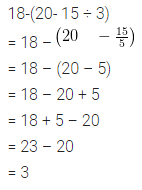Question 2.
-15+ 24÷ (15-13)
Solution: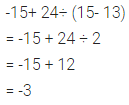Question 3.
35 – [15 + {14-(13 + $$\overline { 2-1+3 }$$)}]
Solution:Question 4.
27- [13 + {4-(8 + 4 – $$\overline { 1+3 }$$)}]
Solution: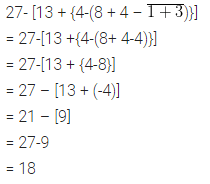Question 5.
32 – [43-{51 -(20 – $$\overline { 18 -7 }$$)}]
Solution:Question 6.
46-[26-{14-(15-4÷ 2 x 2)}]
Solution:Question 7.
45 – [38 – {60 ÷ 3 – (6 – 9 ÷ 3) ÷ 3}]
Solution: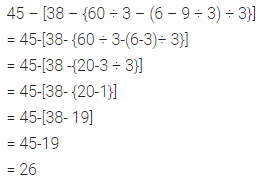Question 8.
17- [17 — {17 — (17 – $$\overline { 17 -17 }$$)}]
Solution:Question 9.
2550 – [510 – {270 – (90 – $$\overline { 80 + 7 }$$)}]
Solution:Question 10.
30+ [{-2 x (25-$$\overline { 13 -3 }$$)}]
Solution:Question 11.
88-{5-(-48)+ (-16)}
Solution: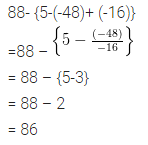Question 12.
9 x (8-$$\overline { 3 +2 }$$) – 2 (2 + $$\overline { 3 +3 }$$)
Solution:Question 13.
2 – [3 – {6 – (5 – $$\overline { 4 -3 }$$)}]
Solution: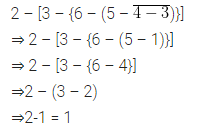### Integers Exercise 1D – Selina Concise Mathematics Class 7 ICSE Solutions

Question 1.
The sum of two integers is -15. If one of them is 9, find the other.
Solution:Question 2.
The difference between an integer and -6 is -5. Find the values of x.
Solution:Question 3.
The sum of two integers is 28. If one integer is -45, find the other.
Solution: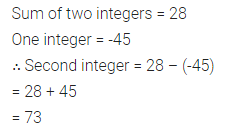Question 4.
The sum of two integers is -56. If one integer is -42, find the other.
Solution: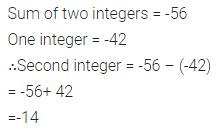Question 5.
The difference between an integer x and (-9) is 6. Find all possible values ofx.
Solution:Question 6.
Evaluate:

1. (-1) x (-1) x (-1) x  ….60 times.
2. (-1) x (-1) x (-1) x (-1) x …. 75 times.

Solution:Question 7.
Evaluate:

1. (-2) x (-3) x (-4) x (-5) X (-6)
2. (-3) x (-6) x (-9) x (-12)
3. (-11) x (-15) + (-11) x (-25)
4. 10 x (-12) + 5 x (-12)

Solution: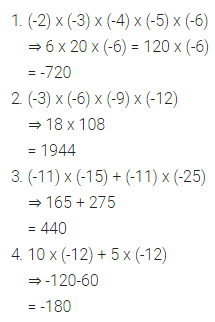Question 8.

1. If x x (-1) = -36, is x positive or negative?
2. If x x (-1) = 36, is x positive or negative?

Solution: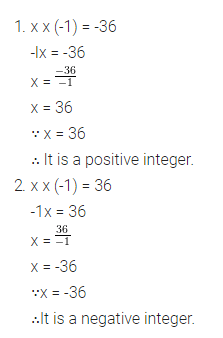∴ It is a positive integer.

Question 9.
Write all the integers between -15 and 15, which are divisible by 2 and 3.
Solution:Question 10.
Write all the integers between -5 and 5, which are divisible by 2 or 3.
Solution: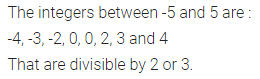Question 11.
Evaluate:

1. (-20) + (-8) ÷ (-2) x 3
2. (-5) – (-48) ÷ (-16) + (-2) x 6
3. 16 + 8 ÷ 4- 2 x 3
4. 16 ÷ 8 x 4 – 2 x 3
5. 27 – [5 + {28 – (29 – 7)}]
6. 48 – [18 – {16 – (5 – $$\overline { 4 +1 }$$)}]
7. -8 – {-6 (9 – 11) + 18 = -3}
8. (24 ÷ $$\overline { 12 -9 }$$ – 12) – (3 x 8 ÷ 4 + 1)

Solution: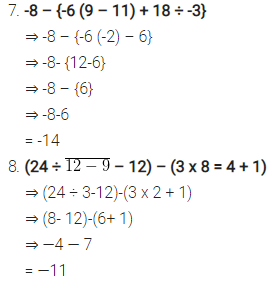Question 12.
Find the result of subtracting the sum of all integers between 20 and 30 from the sum of all integers from 20 to 30.
Solution:Question 13.
Add the product of (-13) and (-17) to the quotient of (-187) and 11.
Solution: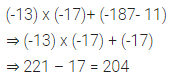Question 14.
The product of two integers is-180. If one of them is 12, find the other.
Solution: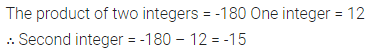Question 15.

1. A number changes from -20 to 30. What is the increase or decrease in the number?
2. A number changes from 40 to -30. What is the increase or decrease in the number?

Solution: